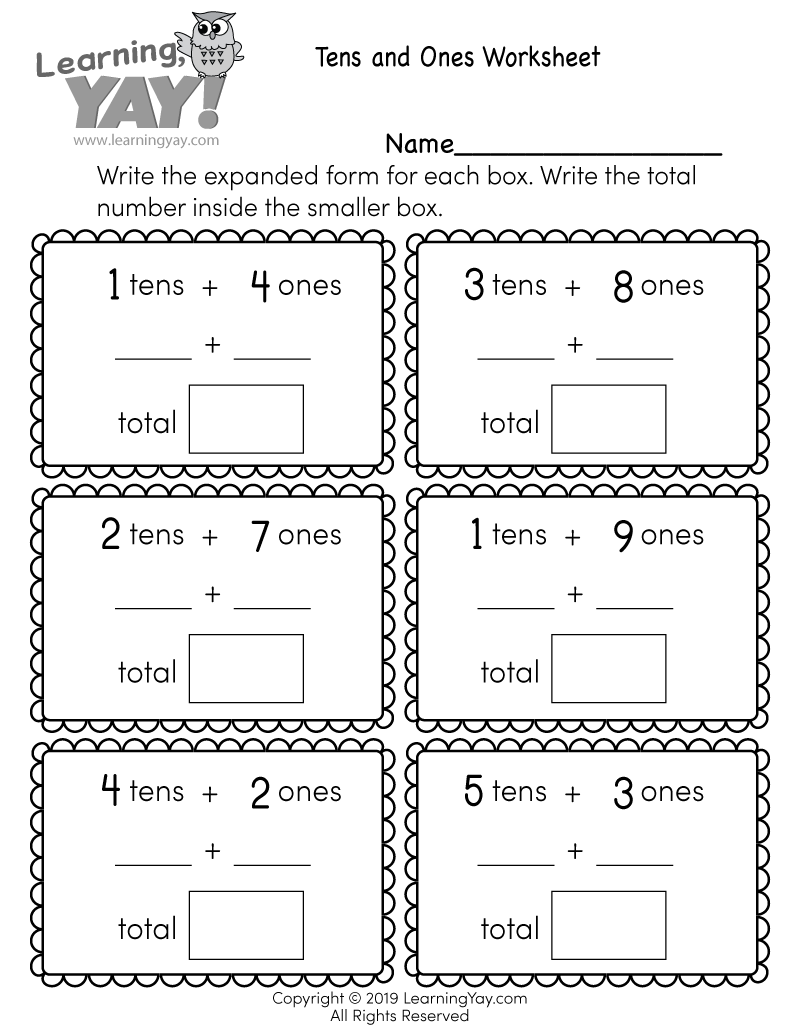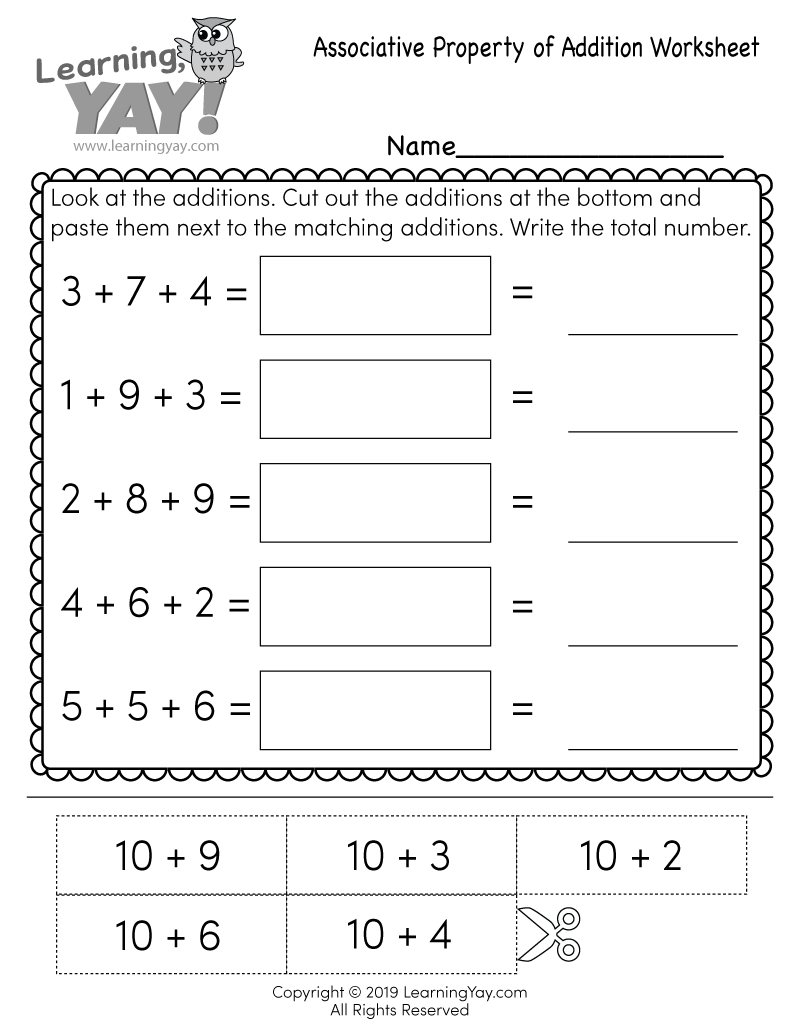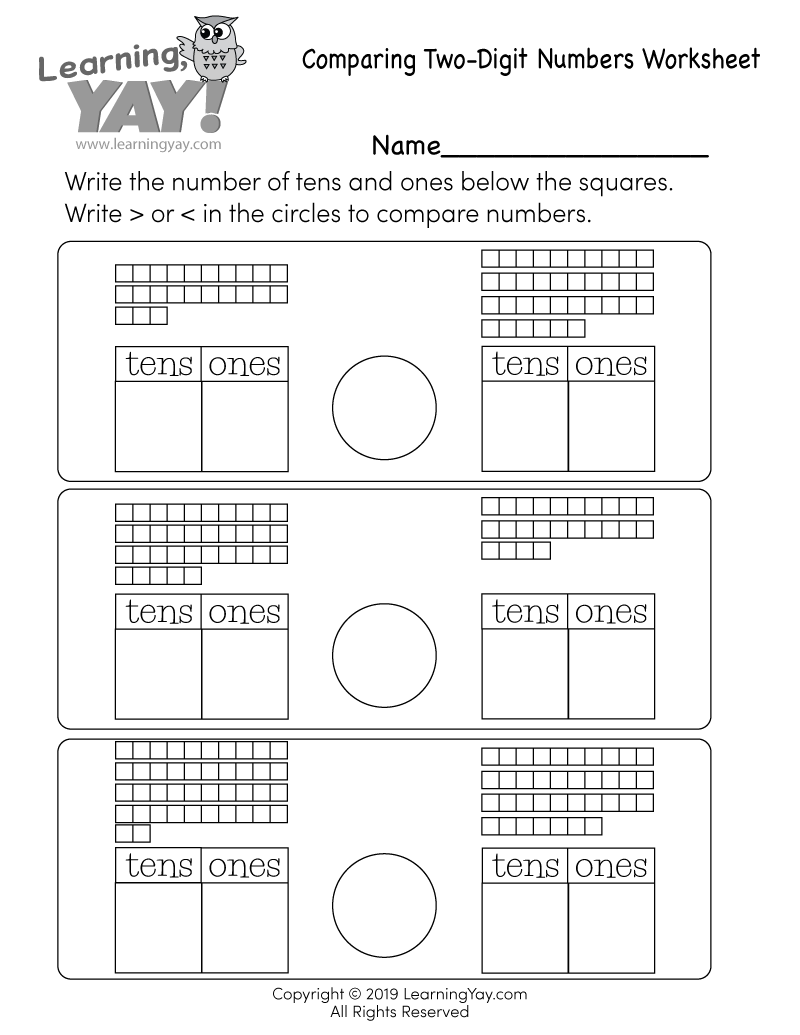# Tens and Ones Worksheet

First graders can improve their understanding that the two digits of a two-digit number represent amounts of tens and ones. Students are asked to write the expanded form of two numbers and then determine the total amount in this free printable place value worksheet.This free printable math worksheet is aligned to first grade Common Core standards. It is an excellent resource for teachers and parents who are teaching the following standards.
##### 1.NBT.B.2 - Common Core ID

Understand that the two digits of a two-digit number represent amounts of tens and ones. Understand the following as special cases:

##### 1.NBT.B.2b - Common Core ID

The numbers from 11 to 19 are composed of a ten and one, two, three, four, five, six, seven, eight, or nine ones.

Common Core » 1st Grade Math Standards » Number & Operations in Base Ten » Understand place value. » 1.NBT.B.2, 1.NBT.B.2b

## There are multiple ways to get this worksheet.

### You might also like these math worksheets: